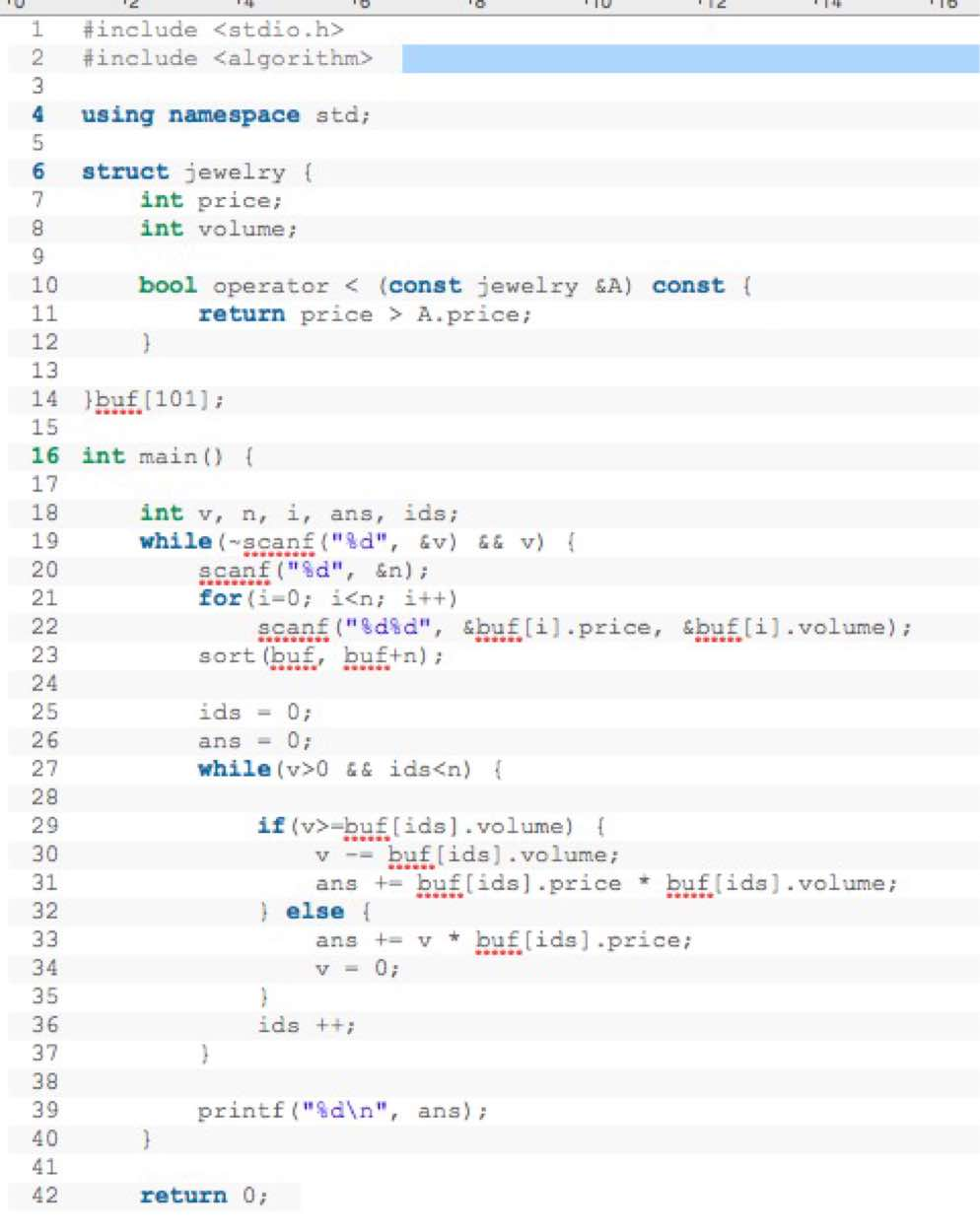#include

#include

using namespace std;

struct jewelry {

int price;

int volume;

bool operator < (const jewelry &A) const {

return price > A.price;

}

}buf;

int main() {

int v, n, i, ans, ids;

while(~scanf("%d", &v) && v) {

scanf("%d", &n);

for(i=0; i scanf("%d%d", &buf[i].price, &buf[i].volume);
sort(buf, buf+n);
ids = 0;
ans = 0;
while(v>0 && ids if(v>=buf[ids].volume) {

v -= buf[ids].volume;

ans += buf[ids].price * buf[ids].volume;

} else {

ans += v * buf[ids].price;

v = 0;

}

ids ++;

}

printf("%d\n", ans);

}

return 0;5个回答

`````` class jewelry {
public int price;
public int volume;
boolean compareto (jewelry A) {
return this.price > A.price;
}
static jewelry[] buf = new jewelry;
void main() {
int v, n, i, ans, ids;
Scanner in = new Scanner(System.in);
while(in.hasNextInt()) {
v = in.nextInt();
n =  in.nextInt();
for(i=0; i<n; i++)
{
buf[i] = new jewelry();
buf[i].price= in.nextInt();
buf[i].volume = in.nextInt();
}
sort(buf, buf+n);
ids = 0;
ans = 0;
while(v>0 && ids<n) {
if(v>=buf[ids].volume) {
v -= buf[ids].volume;
ans += buf[ids].price * buf[ids].volume;
} else {
ans += v * buf[ids].price;
v = 0;
}
ids++;
}
System.out.println(ans);
}
return 0;
}
``````

----------------------Hi，地球人，我是问答机器人小S，上面的内容就是我狂拽酷炫叼炸天的答案，除了赞同，你还有别的选择吗？esc_ai 回复dyxm21: 我是说题目在哪里，要做什么？dyxm21 回复ypblog: 这个是一道acm题目，只有c语言的没有java的

java导入包 ，导入源文件：
package org.noc,,,,
import java.io,,,,,

java main：
public static void main(args []){
}

scanf = println

``````    public static void sort(jewelry[], int n)
{
for (int i = 0; i < n - 1; i++)
{
for (int j = 0; j < n - 1; j++)
{
if (jewelry[j].compareto[j + 1])
{
jewelry t = jewelry[j];
jewelry[j] = jewelry[j + 1];
jewelry[j + 1] = t;
}
}
}
}
``````